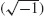# Mathematical Introduction

1. Numbers, Exponents, and Logarithms

2. Mathematical Progressions

3. Statistical Measures

Unit I Summary

List of Formulas

Exercises for Unit I

 1 Numbers, Exponents, and Logarithms

## 1.1. NUMBERS

It is essential to understand numbers and their fractions and decimals in finance. Interest rates, time, and financial ratios are commonly expressed by fractions and decimals, and that is where most of the common mistakes are made, especially when it comes to working out computational problems. The common numbers we normally see and use, such as 3, −5, and 0, are called whole numbers or integers (see Figure 1.1). Along with their partials or fractions, they form what are called rational numbers. Conversely, irrational numbers are those numbers that cannot terminate or repeat, such asand e. Both rational and irrational numbers are called real numbers and stand opposite to unreal or imaginary numbers, such as the square root of a negative 1and its multiples, such as.

## 1.2. FRACTIONS

A fraction is a part of a whole, expressed as a numerator (the number on top of a division line) divided by a denominator (the number on the bottom). A fraction such as

Get Mathematical Finance now with the O’Reilly learning platform.

O’Reilly members experience books, live events, courses curated by job role, and more from O’Reilly and nearly 200 top publishers.